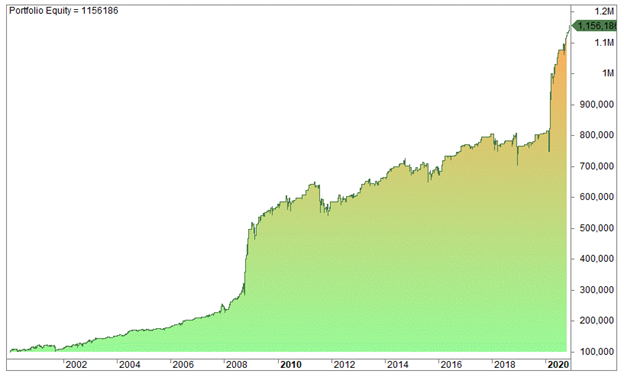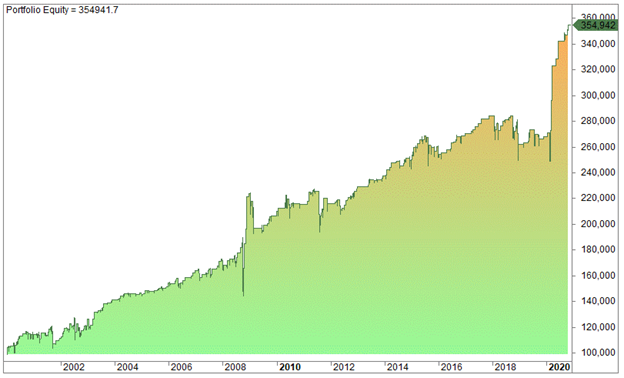Last Updated on 7 September, 2021 by Samuelsson

There are many technical indicators available to traders, but only a few can match the Williams %R indicator when used correctly. In this article, we’ll explain the Williams %R indicator and take a look at its backtested result.

## The Williams %R indicator: what is it?

The Williams %R is an oscillator developed by Larry Williams. It oscillates between 0 to -100 and reflects the current close relative to the highest high for the lookback period. Williams %R is somewhat similar to the stochastic indicator. But stochastic measures the close relative to the lowest low, while Williams %R compares the close to the highest high. A reading above -20 is considered overbought, while a low reading below -80 is considered oversold. The indicator is mainly used for mean-reversion trading.

See how a 5-period Williams %R looks in the SPDR S&P 500 ETF:## How the Williams %R indicator is calculated

Using a 14-period lookback, the formula looks like this:

Williams %R = [(highest high last 14 periods – close) / (highest high last 14 periods – lowest low last 14 periods)] x (-100)

The indicator is best used on the daily timeframe, so the period is in days. So, assuming today’s close is 400, the highest high over the last 14 days was 420, and the lowest low over the last 14 days was 370, then the Williams %R would be {(20/50)x (-100)} = -40.

Does the Williams %R indicator work?

Let’s take a look at a backtesting result done on the S&P 500. The strategy’s entry is on the day’s close when the Williams %R is below -90, while the exit is when the day’s close is higher than the preceding day’s high or when the Williams %R closes above -30.

Optimization showed that the best results on short lookback periods, after testing with a minimum lookback period of two days and a maximum of 25 days. All tests gave a profit factor of two or more, except for 25 days lookback period which produced 1.9. A two-day lookback period gave the best result, and the equity curve is seen below:From the equity curve, you can see that the strategy performed extremely well during the financial crisis of 2008/09 and the 2020 Covid-19 pandemic. The percentage return in 2008 and 2020 were 98.9% and 43.3% respectively! The returns above are compounded, and the key deductions are as follows:

• CAGR is 12.4% (buy and hold is 6.5%)
• Exposure is 23%
• The number of trades is 397
• The average gain per trade is 0.64%
• Max drawdown is 17%
• The profit factor is 2.36

As you can see, these are pretty good numbers, but it’s just backtesting results done on the Amibroker platform. You may be wondering whether the Williams %R works for shorts as well. The truth is, it’s much more difficult to find profitable strategies on the short side.

Comparing Williams %R to RSI

As with the Relative Strength Indicator (RSI), the Williams %R is a mean-reverting indicator. Testing the RSI using the corresponding criteria — enter on the close when the two-day RSI is below 10 and exit on the close when the close is higher than yesterday’s high or when the RSI(2) ends higher than 70 — the equity curve looks like this:The CAGR for the RSI strategy is substantially lower than that for the Williams %R: 6.23% vs. 12.4%. However, this difference could be entirely due to chance and randomness.

Final words

The Williams %R performs quite well in backtests. On a wide range of backtests, it seems to perform better than both the RSI.